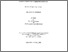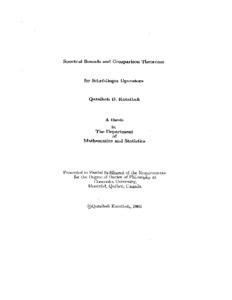Title:

# Spectral bounds and comparison theorems for Schrödinger operators

Katatbeh, Qutaibeh D (2003) Spectral bounds and comparison theorems for Schrödinger operators. PhD thesis, Concordia University.Preview
Text (application/pdf)
NQ85257.pdf
5MB

## Abstract

One of the most important problems in quantum physics is to find the energy eigenvalues for Schrödinger's equation. This equation is exactly solvable only for a small class of potentials. For one-particle problems numerical solutions can always be obtained, but in the absence of exact solutions, the next best thing is an analytical formula for an approximation, such as energy bound. In this thesis we use geometrical techniques such as the envelope method to obtain analytical spectral bounds for Schrödinger's equation for wide classes of potential. Our geometrical approach leans heavily on the comparison theorem, to the effect that V 1 < V 2 [implies] E 1 < E 2 . For the bottom of an angular-momentum subspace it is possible to generalize the comparison theorem by allowing the comparison potentials V 1 and V 2 to cross over in a controlled way and still imply spectral ordering E 1 < E 2 . We prove and use these theorems to sharper some earlier upper and lower bounds obtained using the 'envelope method'. In chapter two we introduce the envelope method that is used in the subsequent chapters. In chapter three we study the Hellmann potential in quantum physics: we prove that discrete eigenvalues exist and we obtain formulae for upper and lower bounds to them. In chapter four, we prove the existence of a discrete spectrum for the cutoff-Coulomb potential, and we obtain upper and lower-bound formulae. In chapter five we prove the monotonicity of the wave function |( r ) for the ground state in the case of attractive central potentials in N N spatial dimensions. By using this result we establish some generalized comparison theorems in which the comparison potentials intersect. We use these theorems, together with the sum approximation, to improve the upper and the lower bounds obtained earlier with the aid of the envelope method. In chapter six, we study the representation P ( q ) for the eigenvalues E ( q ) of the operator H = -x + sgn(q)r q defined by E ( q ) = [Special characters omitted.] . It had earlier been proved that P ( q ) is monotone increasing. We strengthen this result for the ground state (and the bottom of each angular-momentum subspace) by using the generalized comparison theorems to prove that a new function Q ( q ) = Z ( q ) P ( q ) is monotone increasing, where the factor Z ( q ) is monotone decreasing. Thus we know that P ( q ) cannot increase too slowly: this in turn allows us to obtain same improved bounds for the eigenvalues E ( q ) in N dimensions. In the last chapter we analyse bounds for the Coulomb plus power-law potentials obtained by variational methods and the sum approximation.

Divisions: Concordia University > Faculty of Arts and Science > Mathematics and Statistics Thesis (PhD) Katatbeh, Qutaibeh D xiv, 121,  leaves ; 29 cm. Concordia University Ph. D. Mathematics 2003 Hall, Richard L. QC 174.17 S3K38 2003 2209 Concordia University Library 27 Aug 2009 17:26 13 Jul 2020 19:51 https://concordiauniversity.on.worldcat....
All items in Spectrum are protected by copyright, with all rights reserved. The use of items is governed by Spectrum's terms of access.

Repository Staff Only: item control pageResearch related to the current document (at the CORE website)
Back to top